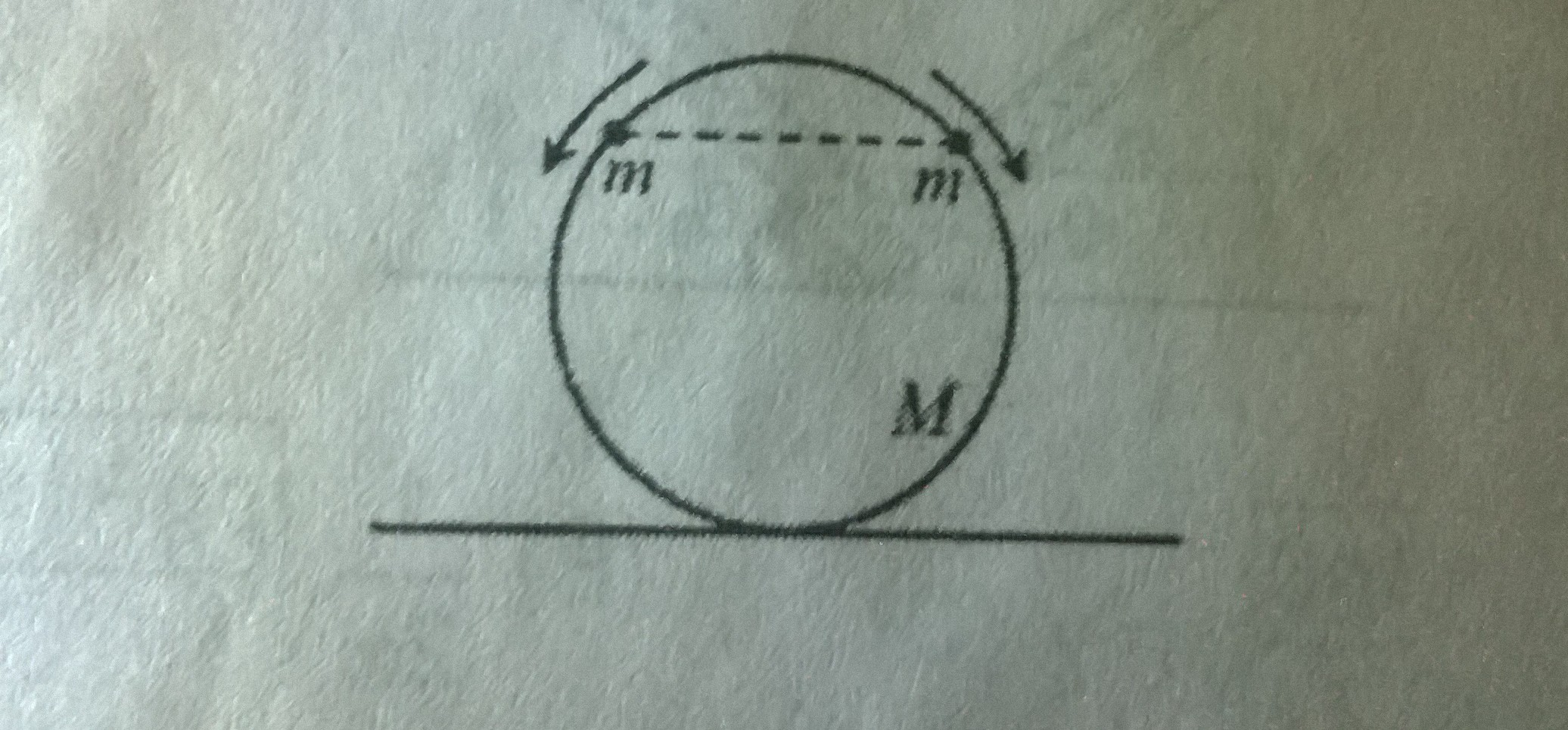# Is it possible?Two beads of mass $m$ each are positioned at the top of a frictionless hoop of mass $M$ with radius $R$ , which stands vertically on the ground. The beads are given a slight push, and they slide down the hoop, one to the right and the other one to the left, as shown . What is the smallest value of $\frac{m}{M}$ for which the hoop will rise up off the ground at some time during the motion?

×